Summability multipliers

Numerical factors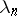(for the terms of a series) that transform a series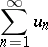(1)

which is summable by a summation method(cf. Summation methods) into a series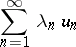(2)

which is summable by a method. In this case, the summability multipliersare called summability multipliers of type. For example, the numbers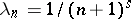are summability multipliers of type(see Cesàro summation methods) when(see ).

The fundamental problem in the theory of summability multipliers is to find conditions under which numberswill be summability multipliers of one type or another. This question is formulated more exactly in the following way: Ifandare two classes of series, then what conditions have to be imposed on the numbersso that for every series (1) from, the series (2) belongs to? The appearance of the theory of summability multipliers goes back to the Dedekind–Hadamard theorem: The series (2) converges for any convergent series (1) if and only ifwhere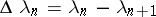. There is a generalization of this theorem with summability by the Cesàro method.